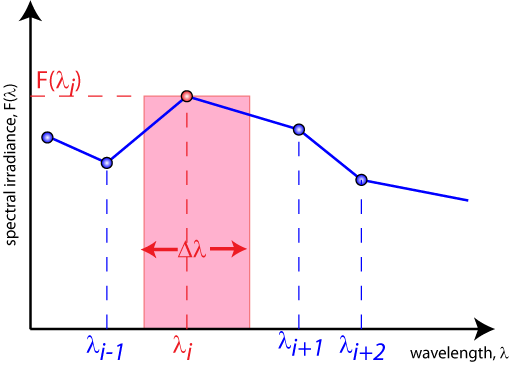# 辐射功率密度

$H={\int }_{0}^{\infty }F\left(\lambda \right)d\lambda$

H是光源发出的总功率密度单位是W m-2

F(λ)是光谱辐照度，单位是Wm-2μm-1

$H=\sum _{i}F\left(\lambda \right)\text{Δ}\lambda$

H是光源发出的总功率密度单位是W m-2

F(λ) 是光谱辐照度，单位是Wm-2μm-1

Δλ是波长要计算某个光源的总功率密度，需要计算出每个单元的面积，然后将它们加在一起，在光谱上进行积分。

$\begin{array}{c}\Delta \lambda =\frac{{\lambda }_{i+1}+{\lambda }_{i}}{2}-\frac{{\lambda }_{i}+{\lambda }_{i-1}}{2}\\ =\frac{{\lambda }_{i+1}-{\lambda }_{i-1}}{2}\end{array}$

${H}_{i}=\Delta \lambda \cdot F\left({\lambda }_{i}\right)$